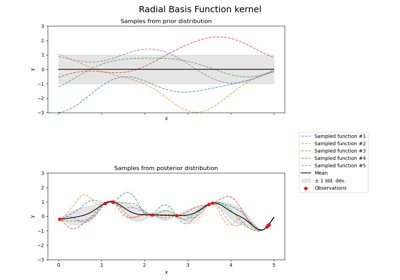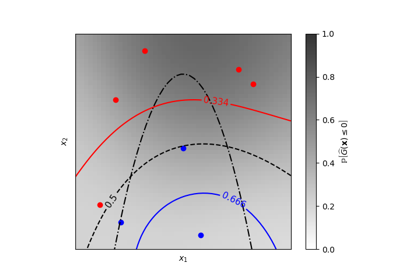# sklearn.gaussian_process.kernels.ConstantKernel¶

class sklearn.gaussian_process.kernels.ConstantKernel(constant_value=1.0, constant_value_bounds=(1e-05, 100000.0))[source]

Constant kernel.

Can be used as part of a product-kernel where it scales the magnitude of the other factor (kernel) or as part of a sum-kernel, where it modifies the mean of the Gaussian process.

$k(x_1, x_2) = constant\_value \;\forall\; x_1, x_2$

kernel = RBF() + ConstantKernel(constant_value=2)


is the same as:

kernel = RBF() + 2


Read more in the User Guide.

New in version 0.18.

Parameters:
constant_valuefloat, default=1.0

The constant value which defines the covariance: k(x_1, x_2) = constant_value

constant_value_boundspair of floats >= 0 or “fixed”, default=(1e-5, 1e5)

The lower and upper bound on constant_value. If set to “fixed”, constant_value cannot be changed during hyperparameter tuning.

Attributes:
bounds

Returns the log-transformed bounds on the theta.

hyperparameter_constant_value
hyperparameters

Returns a list of all hyperparameter specifications.

n_dims

Returns the number of non-fixed hyperparameters of the kernel.

requires_vector_input

Whether the kernel works only on fixed-length feature vectors.

theta

Returns the (flattened, log-transformed) non-fixed hyperparameters.

Examples

>>> from sklearn.datasets import make_friedman2
>>> from sklearn.gaussian_process import GaussianProcessRegressor
>>> from sklearn.gaussian_process.kernels import RBF, ConstantKernel
>>> X, y = make_friedman2(n_samples=500, noise=0, random_state=0)
>>> kernel = RBF() + ConstantKernel(constant_value=2)
>>> gpr = GaussianProcessRegressor(kernel=kernel, alpha=5,
...         random_state=0).fit(X, y)
>>> gpr.score(X, y)
0.3696...
>>> gpr.predict(X[:1,:], return_std=True)
(array([606.1...]), array([0.24...]))


Methods

 __call__(X[, Y, eval_gradient]) Return the kernel k(X, Y) and optionally its gradient. clone_with_theta(theta) Returns a clone of self with given hyperparameters theta. Returns the diagonal of the kernel k(X, X). get_params([deep]) Get parameters of this kernel. Returns whether the kernel is stationary. set_params(**params) Set the parameters of this kernel.

Return the kernel k(X, Y) and optionally its gradient.

Parameters:
Xarray-like of shape (n_samples_X, n_features) or list of object

Left argument of the returned kernel k(X, Y)

Yarray-like of shape (n_samples_X, n_features) or list of object, default=None

Right argument of the returned kernel k(X, Y). If None, k(X, X) is evaluated instead.

Determines whether the gradient with respect to the log of the kernel hyperparameter is computed. Only supported when Y is None.

Returns:
Kndarray of shape (n_samples_X, n_samples_Y)

Kernel k(X, Y)

K_gradientndarray of shape (n_samples_X, n_samples_X, n_dims), optional

The gradient of the kernel k(X, X) with respect to the log of the hyperparameter of the kernel. Only returned when eval_gradient is True.

property bounds

Returns the log-transformed bounds on the theta.

Returns:
boundsndarray of shape (n_dims, 2)

The log-transformed bounds on the kernel’s hyperparameters theta

clone_with_theta(theta)[source]

Returns a clone of self with given hyperparameters theta.

Parameters:
thetandarray of shape (n_dims,)

The hyperparameters

diag(X)[source]

Returns the diagonal of the kernel k(X, X).

The result of this method is identical to np.diag(self(X)); however, it can be evaluated more efficiently since only the diagonal is evaluated.

Parameters:
Xarray-like of shape (n_samples_X, n_features) or list of object

Argument to the kernel.

Returns:
K_diagndarray of shape (n_samples_X,)

Diagonal of kernel k(X, X)

get_params(deep=True)[source]

Get parameters of this kernel.

Parameters:
deepbool, default=True

If True, will return the parameters for this estimator and contained subobjects that are estimators.

Returns:
paramsdict

Parameter names mapped to their values.

property hyperparameters

Returns a list of all hyperparameter specifications.

is_stationary()[source]

Returns whether the kernel is stationary.

property n_dims

Returns the number of non-fixed hyperparameters of the kernel.

property requires_vector_input

Whether the kernel works only on fixed-length feature vectors.

set_params(**params)[source]

Set the parameters of this kernel.

The method works on simple kernels as well as on nested kernels. The latter have parameters of the form <component>__<parameter> so that it’s possible to update each component of a nested object.

Returns:
self
property theta

Returns the (flattened, log-transformed) non-fixed hyperparameters.

Note that theta are typically the log-transformed values of the kernel’s hyperparameters as this representation of the search space is more amenable for hyperparameter search, as hyperparameters like length-scales naturally live on a log-scale.

Returns:
thetandarray of shape (n_dims,)

The non-fixed, log-transformed hyperparameters of the kernel

## Examples using sklearn.gaussian_process.kernels.ConstantKernel¶Illustration of prior and posterior Gaussian process for different kernels

Illustration of prior and posterior Gaussian process for different kernelsIso-probability lines for Gaussian Processes classification (GPC)

Iso-probability lines for Gaussian Processes classification (GPC)